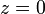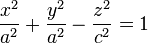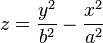# Difference between revisions of "Doubly ruled surface"

This article defines a property that makes sense for a surface embedded in$\R^3$, viz three-dimensional Euclidean space. The property is invariant under orthogonal transformations and scaling, i.e., under all similarity transformations.
View other such properties

## Definition

A surface embedded in$\R^3$ is said to be doubly ruled if given any point in the surface, there are two distinct lines through the point, that lie completely on the surface.

## Classification

Further information: Classification of doubly ruled surfaces

Doubly ruled surface Equational/implicit description
Euclidean plane$z = 0$ (the$xy$-plane)
circular hyperboloid of one sheet$\frac{x^2}{a^2} + \frac{y^2}{a^2} - \frac{z^2}{c^2} = 1$
hyperbolic paraboloid$z = \frac{y^2}{b^2} - \frac{x^2}{a^2}$